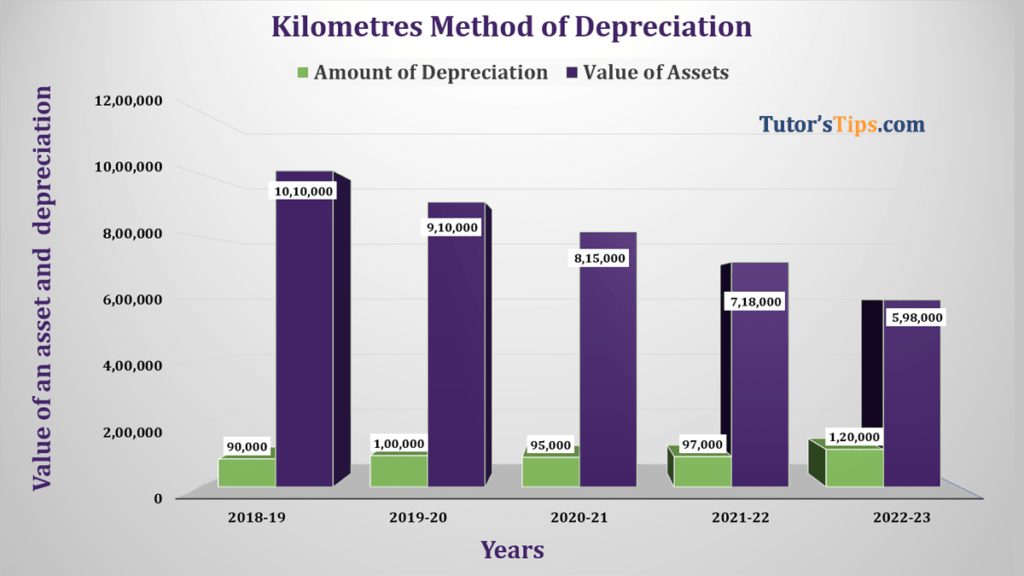# Mileage or Kilometre Method of Depreciation | ExampleMileage or Kilometre Method of Depreciation is very much similar to the Machine hour rate method. But it is applied on the vehicle instead of the machine. So the life of the vehicle can be measured into total kilometres will be covered by it. Rate per mile or kilometres can we determine when the total cost of an asset divided by to kilometres it can run in its whole life. The amount of annual dep will be calculated by multiplying total kilometres run during the financial year by the rate of per kilometre.

### This method of depreciation is applied in the following cases: –

• Where we can calculate the accurate life of an asset in Kilometres.
• Where the life of an asset is certain.

### Example of Mileage or Kilometre Method of Depreciation:

A Truck was purchased on 1st April 2018 at a cost of Rs 11,00,000/- and it can be disposed of for Rs 1,00,000/-. The expected life of truck in Kilometres will be 1,00,000 KM. The following table shows the total running kilometres of the Truck during the particular year in the next 5 years.

1. 31/03/2019 – 9,000
2. 31/03/2020 – 10,000
3. 31/03/2021 – 9,500
4. 31/03/2022 – 9,700
5. 31/03/2023 – 12,000

Calculate the amount of depreciation.

Solution : –

Calculation of amount of depreciation under Mileage or Kilometre Method of Depreciation:-

Formula:-

So now, we will calculate the amount of depreciation with above shown formula:

Total Cost of an asset                   =  10,00,000

Scrap value of an asset                =  1,00,000/-

Total life of an asset(in hours)    =  1,00,000 hours

So,

Depreciation(per kilometre)               =  10,00,000-1,00,000 / 1,00,000

=   Rs. 10 per kilometre

So now, the following table shows the calculation of an amount of depreciation year by year:-

 Year Total working hour (per year) Rate of Depreciation (Per Kilometre) Amount of Depreciation for Year 2018-19 9,000 10 90,000 2019-20 10,000 10 1,00,000 2020-21 9,500 10 95,000 2021-22 9,700 10 97,000 2022-23 12,000 10 1,20,000